Reach Us+44-7482-875032
On the Validity of Modal Expansion in Pekeris Waveguide with PML | OMICS International
Journal of Applied & Computational Mathematics
All submissions of the EM system will be redirected to Online Manuscript Submission System. Authors are requested to submit articles directly to Online Manuscript Submission System of respective journal.

# On the Validity of Modal Expansion in Pekeris Waveguide with PML

Jianxin Zhu* and Zengsi Chen

Department of Mathematics, Zhejiang University, Hangzhou 310027, China

*Corresponding Author:
Jianxin Zhu
Department of Mathematics
Zhejiang University
Hangzhou 310027, China
E-mail: [email protected]

Received January 05, 2013; Accepted January 28, 2013; Published February 06, 2013

Citation: Zhu J, Chen Z (2013) On the Validity of Modal Expansion in Pekeris Waveguide with PML. J Applied Computat Mathemat 2:124. doi: 10.4172/2168-9679.1000124

Copyright: © 2013 Zhu J, et al. This is an open-access article distributed under the terms of the Creative Commons Attribution License, which permits unrestricted use, distribution, and reproduction in any medium, provided the original author and source are credited.

Visit for more related articles at Journal of Applied & Computational Mathematics

#### Abstract

The perfectly matched layer (PML) is a widely used tool to truncate the infinite domain in numerical computations. It can also be used in the modal expansion of an open Pekeris waveguide. In a bounded waveguide with PML, the modal expansion consists of three kinds of modes. They are propagation modes, leaky modes and PML modes. The PML modes are introduced by the utilization of PML, and depend on the parameters of the PML. The validity of the modal expansion of the PML-truncated waveguide is discussed in this paper. It is proved that the expansion coefficient tends to zero when the index of modes tends to infinity, thus the truncation of the infinite sum is reasonable. Moreover, it is also proved that the numerical computation of the coefficients is stable.

#### Keywords

Acoustic waveguide; Perfectly matched layer; Modal expansion; Asymptotic solution method

#### Introduction

In underwater acoustics , the Pekeris waveguide is a simple model, which has been studied extensively . For actual calculation, the region in consideration is always very large, such as the entire ocean, and the problem is usually reduced to a mathematically well-known Helmholtz equation over the infinite domain. The modal expansion of the waveguide consists of a few propagation modes and an integral of continuous radiation modes [3,4], which is difficult to evaluate in practical computations. When the transverse direction is bounded, many methods can be used [5-8]. A perfectly matched layer (PML), introduced by Berenger in 1994 [9,10], is a common tool to truncate the unbounded domain. It is an addition layer around the interested domain, in which the solutions decay. Mathematically, introducing a PML is equivalent to applying a complex coordinate transformation inside the additional layers. Modal expansion of the bounded problem with PML is quite different from the unbounded one. All the modes are discrete, and the integration is approximated by the infinite sum of leaky modes and PML modes. Although the modal expansion method has been used for calculation widely, there are few literatures on the theoretical analysis and numerical verifications, when it combines with PML.

In this paper, we give a discussion on the validity of modal expansion in Pekeris waveguide which is truncated by a PML . Due to the introduction of PML, the coordinate-transformed operator is not self-adjoint, and the eigenfunctions lose the property of orthogonality. In this situation, the coefficients of modal expansion are difficult to evaluate. We use the conjugate eigenfunctions , which are analytical expressed, to compute the expansion coefficients. And the eigenvalues are given by the asymptotic approximation . The theoretical results on the convergency and stability of the coefficients are derived in this paper.

This paper is organized in the following manner. The mathematical formulation of bounded waveguide and the modal expansion method is presented in the section Model and Methods, and the numerical methods and theoretical results on convergency and stability are also given here. Numerical examples are given in the next section. Finally, we leave the conclusions.

#### Materials and Methods

Mathematical model of waveguide

When wave propagates in the Pekeris waveguide , the following two-dimensional Helmholtz equation is considered: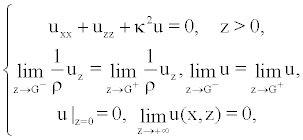(1)

where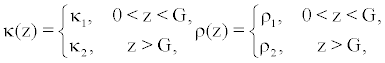and z is the depth, x is the range, k1 and k2 are wave numbers in different fluid layers, ρ1 and ρ1 are densities. For a range-independent waveguide where κ only depends on the depth z, the general solution of (1) has the form u = φ(z)eiβx, in which φ and λ = β2 satisfy the following eigenvalue problem: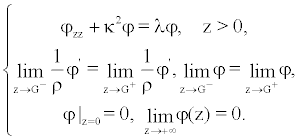(2)

The solution of problem (1) satisfies u ∈ L2 [0,+∞], and it has the modal expansion form: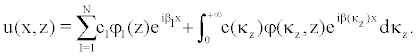(3)

Where =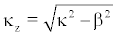, and the eigenfunctions of (2) have the property of weighted orthogonality . However, to slove the Helmholtz equation (1) or further approximations numerically, the depth z must be truncated. As a result, the problem is restricted to a finite interval.

Under the assumption that bottom is homogeneous for z>G, the PML technique is introduced in the depth direction. It is equivalent to a complex coordinate transformation, that is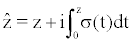. If the interested interval is 0<z<H for some H>G, the PML is added on the boundary x=H, and is terminated at D. For 0 ≤ z ≤ H, σ(z)=0. Defining an operator L by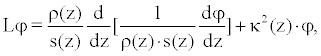the problem (2) is therefore approximated by the following form: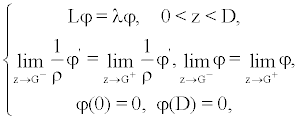(4)

where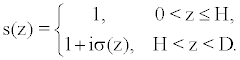The technique described above result in an eigenvalue problem (4) on a bounded interval, whose solutions satisfy u ∈ L2 [0, D]. F. Olyslager has proved that the discrete spectrum of the problem with PML (4) converges to the continuous spectrum of (2) in . As a result, we have the modal expansion form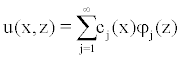(5)

exactly when the thickness of PML tends to infinity. In practical applications, the series must be truncated to a finite sum. In the following sections, we are going to show that the truncation is reasonable by showing the convergency and stability of the coefficients. As the eigenfunctions lose the property of orthogonality, and the determination of coefficients is technical, the method for which is given afterwards.

Eigenfunctions and conjugate eigenfunctions

The eigenfunctions φj (z) (j = 1,2,…) of (4) do not have the property of weighted orthogonality, that brings difficulty in determining the expansion coefficients. Fortunately, the analytical expression of conjugate eigenfunctions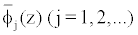can be derived , which are defined by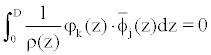, for k ≠ j. Then cj(x) can be expressed analytically as follows: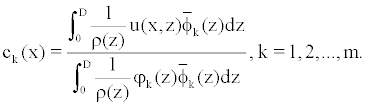(6)

Denoting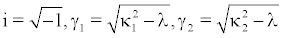, the eigenfunctions can be obtained by solving problem (4) formally, which are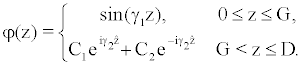(7)

Here, γj (j = 1,2) are chosen to satisfy the imaginary part of γ >, and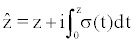. Considering the boundary conditions, the parameters can be determined as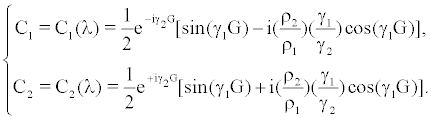The conjugate operator of can be obtained easily by its definition, which is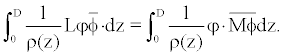The resulting operator M is defined by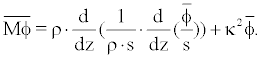In other words,should satisfy the following ODE: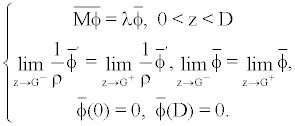(9)

As before, the solutions of equation (9) turn out: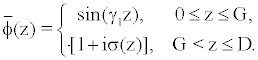(10)

Eigenvalues and expansion coefficients

From the formulas of (6), (7), (10), once the eigenvalues are obtained, the corresponding expansion coefficients can be determined directly. Next, numerical calculation of eigenvalues is discussed.

Although eigenvalue problems are usually difficult to solve, under certain conditions, there exist some good methods. In this paper, asymptotic solution method is used. In order to get accuracy modes, a nonlinear equation for the eigenvalues of this problem is derived, which is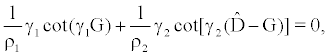(11)

where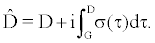The above equation is called dispersion relation, and its deduction process has used the boundary information φ(D)=0 in (4). The roots of (11) corresponding to the eigenvalues of (4).

Using the dispersion relation, Jianxin Zhu et al. [13,15] have given the asymptotic formulas of both PML modes and leaky modes of Pekeris waveguide. Here, we just quote the results as follows:

Leaky modes satisfy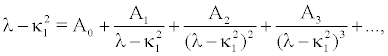which have the following asymptotic formulas: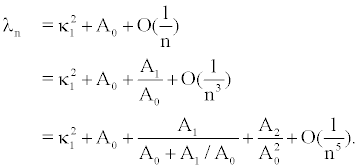(12)

PML modes satisfy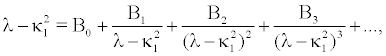whose asymptotic formulas are given similarly: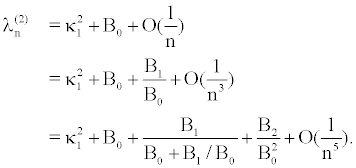(13)

All the parameters mentioned above can be found in .

As mentioned earlier, there is a close relationship between eigenvalues and the corresponding expansion coefficients. Denote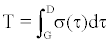, the following theorems can be derived.

Theorem 1: If λ1, λ1, λ1, … are eigenvalues of (4), which ordered by their absolute value in ascending order, and u(x,z) is a continuous function, D > T then when |λk| → ∞, we have |ck| → 0.

The eigenvalues in next theorem are the same to Theorem 1.

Theorem 2: For any eigenvalue λ ∈ {λ1, λ2, λ3,…}, given ε>0, let Dε be a circle with the radius ε at center λ, u(x,z) is a continuous function, and known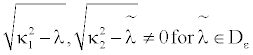, there exist two constant T and N for each λ, such that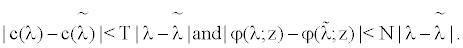We leave the details of proof in APPENDIX. Theorem 1 proves the necessary condition of convergence property for expansion coefficients, and Theorem 2 gives the stability of coefficients when the corresponding eigenvalues are perturbed.

#### Numerical Examples

In order to further illustrate the two Theorems, and verify that ck (x) tends to zero, we first calculate the eigenvalues.

For a numerical example, we consider a Pekeris waveguide with parameters given by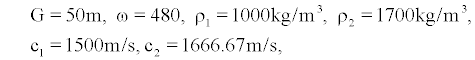where κj= ω/cj for j=1,2. And we use the following parameters for PML: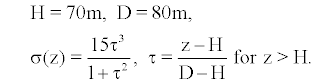The eigenvalues solved by the asymptotic solutions (12) and (13) are shown in Figure 1. The distribution of all eigenvalues is in the left plot, while the local distribution is in the right one.

Figure 1: Eigenvalues are solved by asymptotic solution method, where the whole eigenvalues are plotted in the left, and the local ones are plotted in the right.

As the eigenvalues are calculated, the next step is to calculate the coefficients of the modal expansion. For simplify, the modal expansion of incident wave is considered. We set the incident wave u(0,z)=u0(z), and the coefficients ck (x) are constants marked by ck.

Calculate coefficients

In this example, we choose two incident waves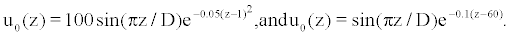After the above calculation, all modes are sorted from small to large according to their imaginary part, and denote their corresponding coefficients as ck, k=1,2,…. Figures 2 and 3 give the absolute values of coefficients in their left. Meanwhile, we adopt a uniform sampling in accordance to the order of k when the first incident wave is considered, and list 10 coefficients, as shown in Table 1.

k λk real(ck) imag(ck)
1 0.0998 0.0719 0
11 -0.2537+0.0155i 0.1027 -0.0084
21 -1.2486+0.0311i -0.0052 0
31 -3.1041+0.0482i -3.5019e-06 0
41 -5.4491+0.0635i -1.4725e-12 0
51 -8.8047+0.0805i -1.2820e-18 0

Table 1: Sample coefficients for u0 (z) = 100sin(π z / D)e-0.05(z-1)2

Figure 2: Coefficients of the modal expansion for u0 (z) = 100sin(π z / D)e-0.05(z-1)2 are plotted in the left, and the comparison of numerical solutions and incident wave is plotted in the right.

Figure 3: Coefficients of the modal expansion for u0 (z) = 100sin(π z / D)e-0.01(z-60) are plotted in the left, and the comparison of numerical solutions and incident wave is plotted in the right.

Next, we give error estimations for modal expansion method applying to these incident waves. The number of expansion is denoted by n. From Tables 2 and 3, we find that the errors will be reduced if we increase the number of terms, but when a certain number of terms reached, the accuracy will not increase evidently. This is due to the fact that the latter coefficients in the expansion are very small and they do little effect. Further more, the cumulative error will also be increased with the increasing of terms. That is to say, we can express u0 by only a few terms. We also get additional information from the data above, considerable part of the errors are concentrated in the PML part, but not in the interested region. What's more, we can see that the approximations work well when only about twenty eigenvalues are used. This shows that the calculated eigenvalues are very close to the exact eigenvalues, and it also further validates Theorem 2. In order to have an intuitive understanding, the comparisons of numerical solutions and incident waves are plotted in the right of Figures 2 and 3. If the incident wave is set by u0 (z) =φ1 (z) or u0 (z) =φ1 (z)+φ2 (z), the expansion coefficients can be obtained as c1 =1, ck= 0(k>1) or c1 =c2 = 1, ck = 0(k> 2) respectively, which grantees the correctness of the algorithm used in this paper.

n Relative error Relative error (exclude PML)
6 0.835201312535519 0.779196561212233
9 0.411655212305537 0.377733421900785
13 0.137896801022559 0.086621018018228
23 0.112271606745862 0.082606001137249
40 0.086537025067915 0.074526842773484

Table 2: Error estimation for modal expansion method, where u0 (z) = 100sin(π z / D)e-0.05(z-1)2

n Relative error Relative error (exclude PML)
6 0.131299559535947 0.097971274304745
9 0.093815612587220 0.055530383686583
13 0.086164084895743 0.049188577856303
23 0.053289890708034 0.041000045633437
35 0.053287041037458 0.040955239573482

Table 3: Error estimation for modal expansion method, where u0 (z) = 100sin(π z / D)e-0.1(z-60).

#### Conclusions

The present study discusses the validity of modal expansion method in Pekeris waveguide with PML. Although the modal expansion method has been used for calculation widely, there are few literatures on the theoretical analysis and numerical verifications, when it combines with PML. The Theorems in this paper are very helpful for numerical applications. Theorem 1 is a necessary condition for the using of modal expansion method, and it plays a guiding role for practical applications. Whereas, Theorem 2 ensures that the coefficient c and eigenfunction ? can still maintain a good stability even under the perturbation of eigenvalue. For these reasons, these theoretical results are essential for the study of the modal expansion method.

#### Acknowledgements

This work was partially supported by the Natural Science Foundation of China (NSFC) under grant (No. 11071217), and the Key Project of the Major Research Plan of the NSFC (No. 91130004).

#### References

Select your language of interest to view the total content in your interested language

### Article Usage

• Total views: 12049
• [From(publication date):
March-2013 - Aug 22, 2019]
• Breakdown by view type
• HTML page views : 8231
• PDF downloads : 3818

## Post your commentCan't read the image? click here to refresh
###### Peer Reviewed Journals

Make the best use of Scientific Research and information from our 700 + peer reviewed, Open Access Journals

International Conferences 2019-20

Meet Inspiring Speakers and Experts at our 3000+ Global Annual Meetings

Top